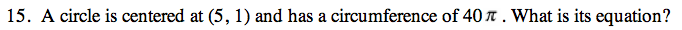13C-15.

### Missing problem for Book PCT => Chapter Ch13 => Lesson 13.1.1 => Problem 13C-15

Created from orphaned homework help problemIf the circumference of a circle is 2πr, what is the radius of this circle?

(xh)2 + (yk)2 = r2